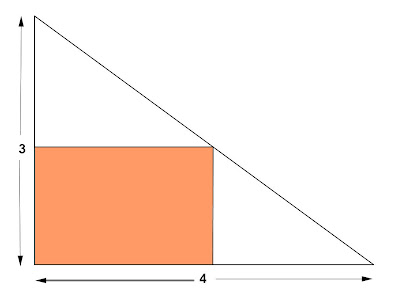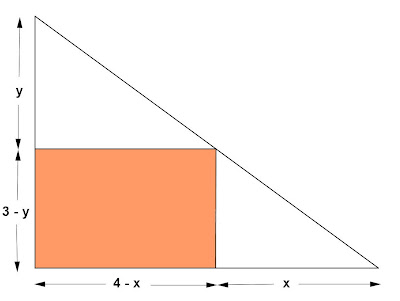## Friday, April 5, 2013

### Maximum Minimum Problem, 4

Category: Differential Calculus, Plane Geometry

"Published in Suisun City, California, USA"

Find the area of the largest rectangle that can be inscribed in a right triangle with legs of lengths 3 cm and 4 cm if two sides of the rectangle lie along the legs as shown in the figure.Photo by Math Principles in Everyday Life

Solution:

Consider the given figure above and label further as followsPhoto by Math Principles in Everyday Life

Area of Rectangle = Length x Width

Next, we need to use similar triangles in order to solve for the value of y as follows

Substitute the value of y to the above equation, we have

Take the derivative on both sides of the equation with respect to x, we have

Set dA/dx = 0 because we want to get the maximum area of the rectangle inscribed in a right triangle as follows

Consider

Substitute the value of x to the above equation, we have

Therefore, the area of the largest rectangle is